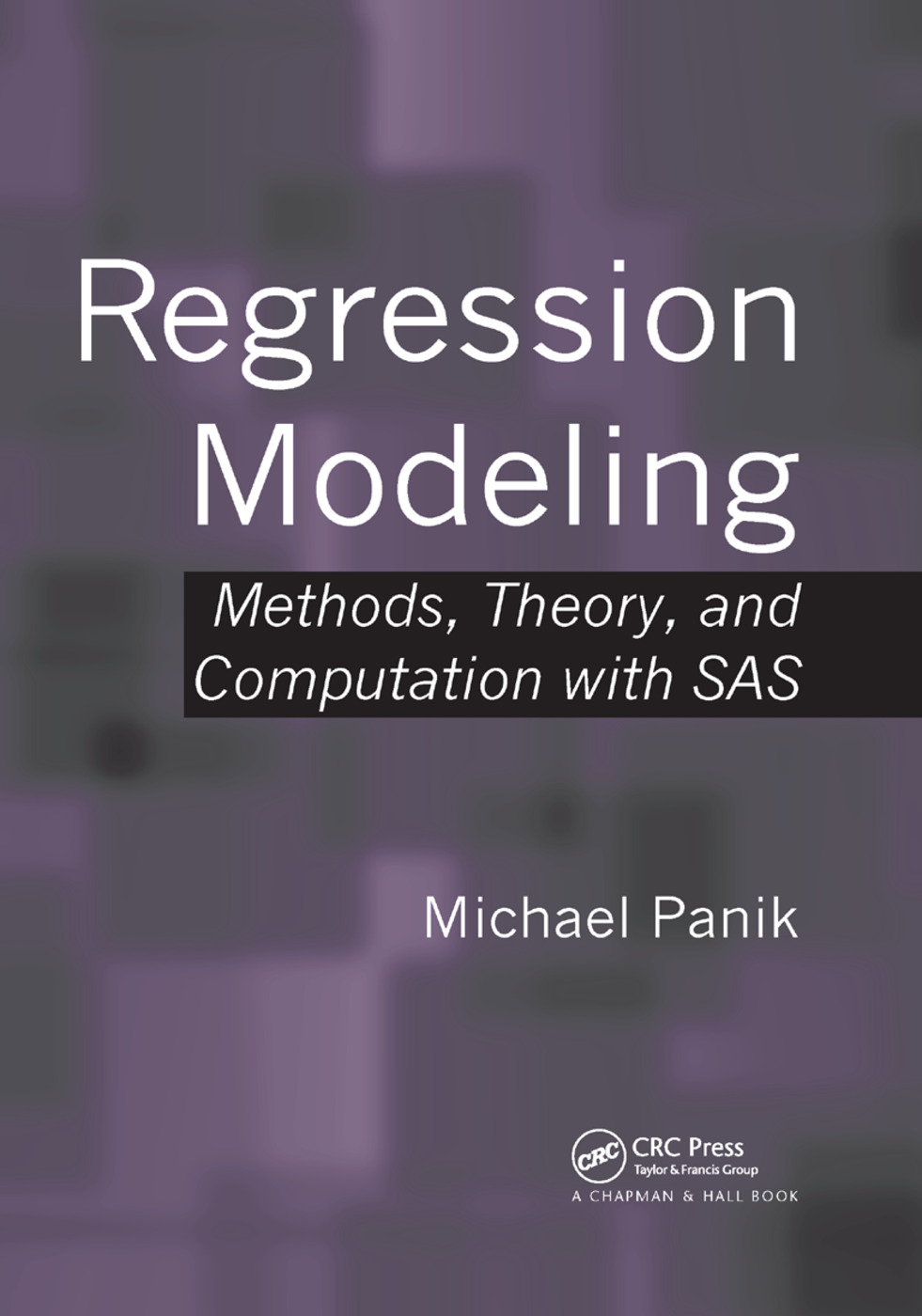# Regression Modeling

## Methods, Theory, and Computation with SAS, 1st Edition

Chapman and Hall/CRC

830 pages | 94 B/W Illus.

Paperback: 9780367385675
pub: 2019-10-07
\$74.95
x
Hardback: 9781420091977
pub: 2009-04-30
\$130.00
x
eBook (VitalSource) : 9780429189036
pub: 2009-04-30
from \$37.48

FREE Standard Shipping!

### Description

Regression Modeling: Methods, Theory, and Computation with SAS provides an introduction to a diverse assortment of regression techniques using SAS to solve a wide variety of regression problems. The author fully documents the SAS programs and thoroughly explains the output produced by the programs.

The text presents the popular ordinary least squares (OLS) approach before introducing many alternative regression methods. It covers nonparametric regression, logistic regression (including Poisson regression), Bayesian regression, robust regression, fuzzy regression, random coefficients regression, L1 and q-quantile regression, regression in a spatial domain, ridge regression, semiparametric regression, nonlinear least squares, and time-series regression issues. For most of the regression methods, the author includes SAS procedure code, enabling readers to promptly perform their own regression runs.

A Comprehensive, Accessible Source on Regression Methodology and Modeling

Requiring only basic knowledge of statistics and calculus, this book discusses how to use regression analysis for decision making and problem solving. It shows readers the power and diversity of regression techniques without overwhelming them with calculations.

### Reviews

This highly readable book should be useful for students, lecturers, and practitioners alike as it covers most of the standard regression techniques and even some methods beyond.

—Karsten Webel, Statistical Papers (2012) 53

As an introductory text, it is mostly successful … . One of the great strengths of the text is that the examples tend to be linked into a structure … so that a student can more easily see how each procedure is connected to the concepts that preceded it. Another strength of the book is the detailed appendices at the end of each chapter. … A unique feature of this book is that it contains many chapters on facets of regression which are not covered in typical introductory texts … the book has great expository strength. It contains detailed verbal descriptions of the procedures used and the reasoning behind them, and these are always clear and linked to the previous descriptions. … the book serves as an excellent conceptual aid to a professor who would prefer to emphasize statistical reasoning to students, rather than to just rely upon the formulaic structure.

The American Statistician, November 2010, Vol. 64, No. 4

In his book, Michael Panik takes up many aspects of modeling with a pedagogical approach, helping the reader to understand the process of the problem and proposed methods. The appendices enrich his process to [readers] who want to increase their knowledge. … this book is a very good tool for students and teachers in statistics, but also for researchers wishing to improve their knowledge in statistical modeling to apply it in their expertise domain.

—Christian Derquenne, Journal of Statistical Software, February 2010

Preface

Review of Fundamentals of Statistics

Bivariate Linear Regression and Correlation

Misspecified Disturbance Terms

Nonparametric Regression

Logistic Regression. Bayesian Regression

Robust Regression. Fuzzy Regression

Random Coefficients Regression

L1 and q-Quantile Regression

Regression in a Spatial Domain

Multiple Regression

Normal Correlation Models

Ridge Regression

Indicator Variables

Polynomial Model Estimation

Semiparametric Regression

Nonlinear Regression

Issues in Time Series Modeling and Estimation

Appendix

References

Index.